Courses

# Stress Distribution In Soil

## 9 Questions MCQ Test Mock Test Series for Civil Engineering (CE) GATE 2020 | Stress Distribution In Soil

Description
This mock test of Stress Distribution In Soil for Civil Engineering (CE) helps you for every Civil Engineering (CE) entrance exam. This contains 9 Multiple Choice Questions for Civil Engineering (CE) Stress Distribution In Soil (mcq) to study with solutions a complete question bank. The solved questions answers in this Stress Distribution In Soil quiz give you a good mix of easy questions and tough questions. Civil Engineering (CE) students definitely take this Stress Distribution In Soil exercise for a better result in the exam. You can find other Stress Distribution In Soil extra questions, long questions & short questions for Civil Engineering (CE) on EduRev as well by searching above.
QUESTION: 1

Solution:
QUESTION: 2

### σz is the vertical stress at a depth equal to z in the soil mass due to a surface point load Q. The vertical stress at depth equal to 2z will be

Solution:

Under the point load, the vertical stress is given by,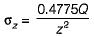(From Boussinesq’s equation at r/z - 0)
∴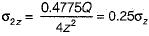QUESTION: 3

### In a Newmark’s influence chart for stress distribution, there are 10 concentric circles and 50 radial lines. The influence factor of the chart is

Solution: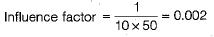QUESTION: 4

Match List-I (Contact pressure distribution diagrams) with List-II (Description of footings) and select the correct answer using the codes given below the lists:
List-I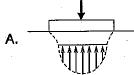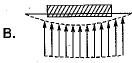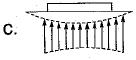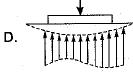List-II
1. Rigid footing on cohesive soil
2. Flexible footing on cohesive soil
3. Rigid footing on cohesionless soil at ground level
4. Flexible footing on cohesionless soil at ground level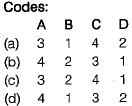Solution:
QUESTION: 5

The intensity of vertical pressure, directly below a concentrated load of 3/2π tonnes at a depth of 3/2π metres is given by

Solution:

According to Boussinesq’s theory,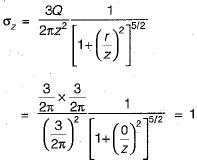QUESTION: 6

Newmark’s influence chart is used for

Solution:
QUESTION: 7

Influence factor ‘I’ depends on

Solution: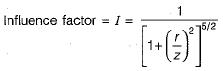QUESTION: 8

Boussinesq’s influence factor for vertical pressure at depth Z and at the centre of a circular area of diameter A carrying uniformly distributed load is

Solution: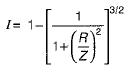∴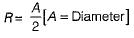∴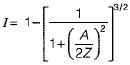QUESTION: 9

Match List-I with List-II and select the correct answer using the codes given below the lists:
List-I
A. Stress distribution due to point load in homogeneous isotropic medium
B. Stress distribution due to point load in an anisotropic soil medium
C. Influence chart for stress distribution in a rectangular area
D. Influence chart for stress distribution in irregularly shaped areas.
List-II
1. Stein Brenner
2. Newmark
3. Boussinesq
4. Westergaard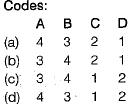Solution: